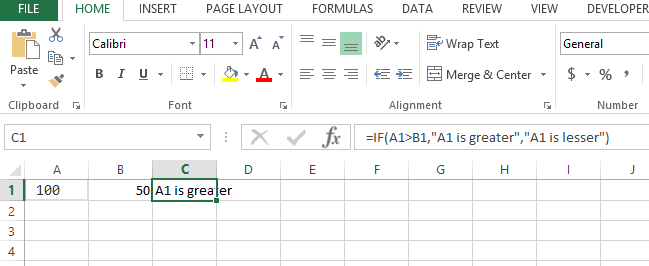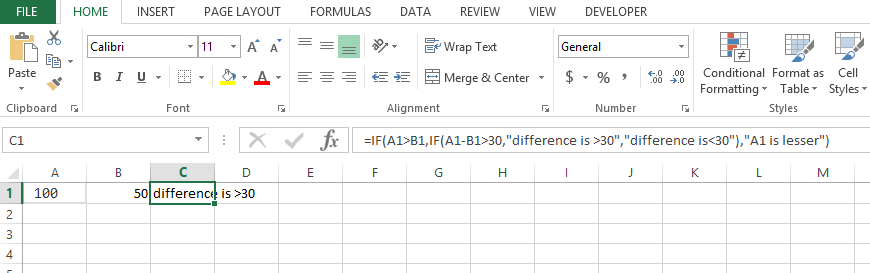### IF Function With Multiple Conditions in Excel

In this article I will guide you to write simple if functions as well as the complex if functions with multiple conditions or we also call it nested if functions. Lets start with simple if function in Excel formulas:

The syntax is like :

=IF( condition, value_if_true, value_if_false)

So there are 3 arguments, first is the condition lik a>b , second is the result if the condition result is true, 3rd argument is the result if the condition is false. e.g:

=IF(A1>B1,”A1 is greater”,”A1 is lesser”)This explains the simple if with one condition but we have more conditions like we also have to check say the difference between the 2 numbers is > 30 of not then we will have to use nested if like:

=IF(A1>B1,IF(A1-B1>30,”difference is >30″,”difference is<30″),”A1 is lesser”)

So we have actually changed the result if true to another if statement like:

=IF(A1-B1>30,”difference is >30″,”difference is<30″)So the result of first condition A1>B1 if it comes true then it will check the next condition

A1-B1>30 and similarly there are 2 results if this condition is true or false.

If we have to write this entire thing in vba code or any coding algorithm it will look like this:

```If A1> B1 Then
If (A1-B1 ) > 30 Then
Msgbox "difference is >30"
Else
Msgbox "difference is <30"
End if
Else
Msgbox “A1 is lesser”
End if```

Based on the code you can see how IF function is nested and how the conditions are being checked.

## Template

```Further reading: